double_doubles - CSDN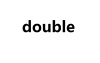double 订阅
double是一个英语单词，意为一对，一双；双重地；酷似的人；把...对折；变成两倍；增加一倍，可作形容词、副词、动词用。 展开全文double是一个英语单词，意为一对，一双；双重地；酷似的人；把...对折；变成两倍；增加一倍，可作形容词、副词、动词用。

double

double单词

• Double 基本数据类型double 的包装类 Double 类型的对象包含一个 double 类型的字段 属性简介 用来以二进制补码形式表示 double 值的比特位数 public static final int SIZE = 64; 二进制补码形式表示 ...
Double 基本数据类型double  的包装类

Double 类型的对象包含一个 double 类型的字段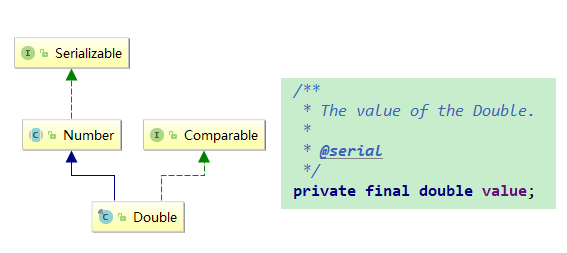属性简介

用来以二进制补码形式表示 double 值的比特位数
public static final int SIZE = 64;
二进制补码形式表示 double 值的字节数
public static final int BYTES = SIZE / Byte.SIZE;
表示基本类型 double 的 Class 实例
public static final Class<Double>   TYPE = (Class<Double>) Class.getPrimitiveClass("double");
能够表示的最大值
只有标准化一种形式,也就是前文提到过的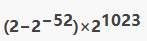public static final double MAX_VALUE = 0x1.fffffffffffffP+1023;
标准化的最小值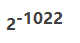public static final double MIN_NORMAL = 0x1.0p-1022;
最小值还有非标准化的形式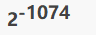public static final double MIN_VALUE = 0x0.0000000000001P-1022;
正无穷
等同于Double.longBitsToDouble(0x7ff0000000000000L)
public static final double POSITIVE_INFINITY = 1.0 / 0.0;
负无穷
等同于Double.longBitsToDouble(0xfff0000000000000L).
public static final double NEGATIVE_INFINITY = -1.0 / 0.0
NaN
not a number
等同于Double.longBitsToDouble(0x7ff8000000000000L)
public static final double NaN = 0.0d / 0.0
指数真值的有效的最大值
public static final int MAX_EXPONENT = 1023
指数真值的有效的最小值
public static final int MIN_EXPONENT = -1022

这些属性,看过浮点数简介的话,可以很清晰的理解,再次说明下,但凡本人的系列文章,全部都是有顺序的

构造方法

Double 依然提供了根据基本类型double以及double的String形式构造

String形式依然借助于parseXXX形式 parseDouble

Double(double value)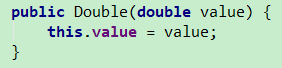Double(String s)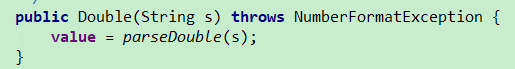常用方法

对于浮点数,有一些额外的属性方法

我们浮点数介绍中,对于浮点数的表示形式进行了介绍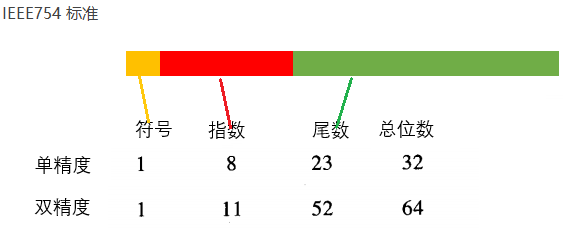Double提供了对于指定值的表示形式的获取方法, 这表示形式也就是是一个64位的二进制位序列

Double 获取表示形式
对于获取表示形式 提供了两种形式的方法,主要是针对于非数字的NaN的不同表示
他们可以与longBitsToDouble 可以进行互相转换
doubleToRawLongBits(double)

根据 IEEE 754 浮点“双精度格式”位布局，返回指定浮点值的表示形式，并保留 NaN 值

如果参数是正无穷大，则结果为 0x7ff0000000000000L

如果参数是负无穷大，则结果为 0xfff0000000000000L

如果参数是 NaN，则结果是表示实际 NaN 值的 long 整数

与 doubleToLongBits 方法不同

doubleToRawLongBits 并没有将 NaN 编码为一个“规范的”NaN 值

在所有情况下，结果都是一个 long 整数

将其赋予 longBitsToDouble(long) 方法将生成一个与 doubleToRawLongBits 的参数相同的浮点值

本地方法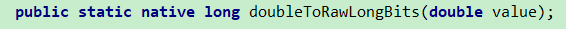doubleToLongBits(double)

根据 IEEE 754 浮点双精度格式 ("double format") 位布局，返回指定浮点值的表示形式

如果参数是正无穷大，则结果为 0x7ff0000000000000L

如果参数是负无穷大，则结果为 0xfff0000000000000L

如果参数是 NaN，则结果为 0x7ff8000000000000L

在所有情况下，结果都是一个 long 整数

将其赋予 longBitsToDouble(long) 方法将生成一个与 doubleToLongBits 的参数相同的浮点值

(所有 NaN 值被压缩成一个“规范”NaN 值时除外）

也就是NaN的处理不一样,此方法将NaN全部规范化为指定固定的值

依赖  doubleToRawLongBits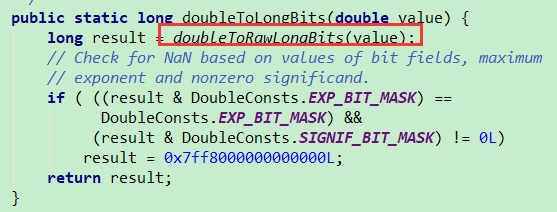longBitsToDouble(long)
返回对应于给定位表示形式的 double 值

如果参数是 0x7ff0000000000000L，则结果为正无穷大

如果参数是 0xfff0000000000000L，则结果为负无穷大

如果参数值在 0x7ff0000000000001L 到 0x7fffffffffffffffL 之间或者在 0xfff0000000000001L 到 0xffffffffffffffffL 之间，则结果为 NaN

浮点数有几种特殊的表示,比如 无穷 NaN等

额外的,也提供了一些相关的方法

static boolean isNaN(double v)
静态方法
是否一个非数字 (NaN) 值
非数值 true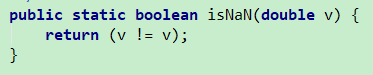static boolean isFinite(double d)
静态方法
是否是有限的浮点数
有限的true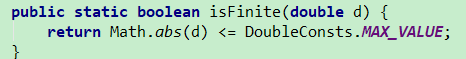static boolean isInfinite(double v)
静态方法
是否是无穷大
是无穷大  true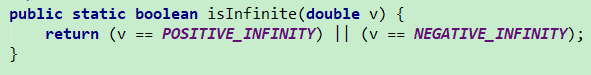boolean isInfinite()
实例方法
依赖静态方法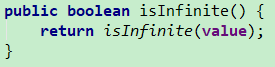boolean isNaN()

实例方法

依赖静态方法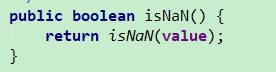比较

static int compare(double d1, double d2)
静态方法
比较两个double
d1 < d2 小于0
d1 = d2 等于0
d1 > d2 大于0
int compareTo(Double anotherDouble)
实例方法
两个对象进行大小比较,依赖于静态方法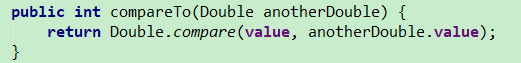parseXXX系列

字符串解析 为 基本类型,

不需要对象,所以都是静态方法返回一个字符串形式表示的基本类型double
表现效果同valueOf(String),不过valueOf 返回的是对象
如果不包含可以解析的字符串将会抛出异常
底层依赖sun.misc.FloatingDecimal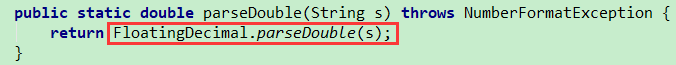valueOf系列

把基本基本类型 包装为对象

用来创建获得对象,所以无需对象,全都是静态方法不同于之前介绍的整数 数值,他们都有缓冲

Double v不存在缓存,valueOf也是直接new 对象

static Double valueOf(double d)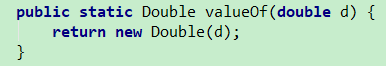static Double valueOf(String s)
依赖parseDouble方法
所以上面说跟valueOf(String)表现效果相同,本身就是一样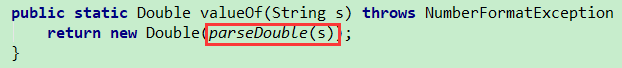Double没有 decode方法

XXXValue系列

类似之前介绍的其他数值类型
全部都是强转内部的  value
return (XXX)value;

byteValue()

shortValue()

intValue()

longValue()

floatValue()

doubleValue()

toString  toXXXString  系列

toString(double)

toString()

toHexString(double)

static String toString(double d)
静态方法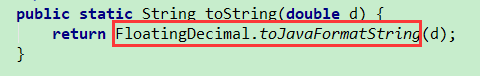String toString()
实例方法
内部调用  static String toString(double d)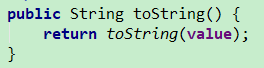static String toHexString(double d)
静态方法
返回 double参数的十六进制字符串表示形式
API帮助文档中对于字符的转换有明确的规定,可以仔细研究下
示例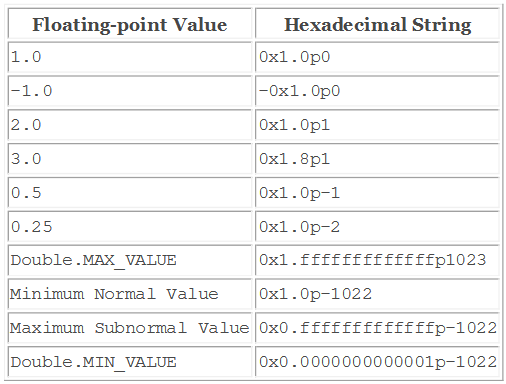toString系列好像没什么好说的,又好像有很多要说的

用到的时候对于格式字符的规定有疑惑直接查看API

equals

boolean equals(Object obj)

将此对象与指定对象比较

当且仅当参数不是 null 而是 Double 对象，且表示的 Double 值与此对象表示的 double 值相同时，结果为 true

为此，当且仅当将方法 doubleToLongBits(double) 应用于两个值所返回的 long 值相同时，才认为这两个 double 值相同

注意，在大多数情况下，对于 Double 类的两个实例 d1 和 d2，当且仅当

d1.doubleValue() == d2.doubleValue()

为 true 时，d1.equals(d2) 的值才为 true

但是，有以下两种例外情况：

如果 d1 和 d2 都表示 Double.NaN，那么即使 Double.NaN==Double.NaN 值为 false，equals 方法也将返回 true

如果 d1 表示 +0.0 而 d2 表示 -0.0，或者相反，那么即使 +0.0==-0.0 值为 true，equals 测试也将返回 false

此定义使得哈希表得以正确操作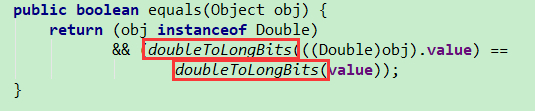hashCode

static int hashCode(double value)
静态方法
获得一个value的hashcode值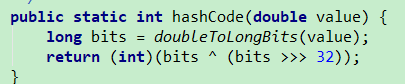int hashCode()
实例方法
依赖静态方法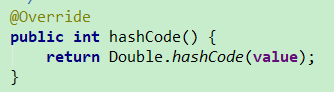其他方法

sum(double, double)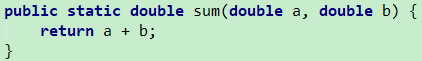max(double, double)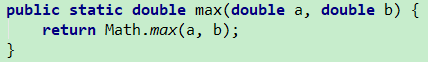min(double, double)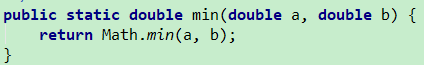总结

其实浮点数的表示形式与使用规范才是重点

就像Float似的, Double只是double的包装,double也只是IEEE754 标准的一个实现,根本还是在于标准的理解

Double  和 Float 提供的方法结构基本上是一样的,毕竟都是浮点数,标准也都是IEEE754

至此,已经介绍了,基本类型包装类中的数值部分

也就是Byte  Short  Integer Long Float Double   他们作为数值有很多类似的方法

这些类似的方法属性也可以说是作为数值类型的共性

个人认为,找到共性能够更好理解运用 java提供的类与功能展开全文• ## Double

2018-04-11 00:54:04
Dubbo功能介绍 Dubbo的发展史 Dubbo是阿里巴巴内部的SOA服务化治理方案的核心框架，每天为2000+ 个服务提供3,000,000,000+ 次访问量支持，并被广泛应用于阿里巴巴集团的各成员站点。Dubbo自2011年开源后，已被...


Dubbo功能介绍

Dubbo的发展史

Dubbo是阿里巴巴内部的SOA服务化治理方案的核心框架，每天为2000+ 个服务提供3,000,000,000+ 次访问量支持，并被广泛应用于阿里巴巴集团的各成员站点。Dubbo自2011年开源后，已被许多非阿里系公司使用。

Dubbo是什么？能做什么？

Dubbo是一个分布式服务框架，以及SOA治理方案。其功能主要包括：高性能NIO通讯及多协议集成，服务动态寻址与路由，软负载均衡与容错，依赖分析与降级等

Dubbo适用于哪些场景？
1：当网站变大后，不可避免的需要拆分应用进行服务化，以提高开发效率，调优性能，节省关键竞争资源等。 2：当服务越来越多时，服务的URL地址信息就会爆炸式增长，配置管理变得非常困难，F5硬件负载均衡器的单点压力也越来越大。 3：当进一步发展，服务间依赖关系变得错踪复杂，甚至分不清哪个应用要在哪个应用之前启动，架构师都不能完整的描述应用的架构关系。 4：接着，服务的调用量越来越大，服务的容量问题就暴露出来，这个服务需要多少机器支撑？什么时候该加机器？等等…… 在遇到这些问题时，都可以用Dubbo来解决。

Dubbo详解
Dubbo是Alibaba开源的分布式服务框架，它最大的特点是按照分层的方式来架构，使用这种方式可以使各个层之间解耦合（或者最大限度地松耦合）。从服务模型的角度来看，Dubbo采用的是一种非常简单的模型，要么是提供方提供服务，要么是消费方消费服务，所以基于这一点可以抽象出服务提供方（Provider）和服务消费方（Consumer）两个角色。关于注册中心、协议支持、服务监控等内容。

如何使用Dubbo

本地服务远程服务Dubbo能做什么？
1：透明化的远程方法调用

-  就像调用本地方法一样调用远程方法
-  只需简单配置，没有任何API侵入
2：软负载均衡及容错机制
-  可在内网代替F5等硬件负载均衡器
3：服务自动注册于发现
-  不在需要写死服务提供方地址，注册中心基于接口名查询服务提供者的IP地址，并可以平滑添加或删除服务提供者。

Dubbo的基本原理展开全文• #include #include #include using namespace std; int main() { cout ************size**************"; cout 所占字节数：" (bool); cout ::max
#include<iostream>
#include<string>
#include <limits>
using namespace std;

int main()
{
cout << "type: \t\t" << "************size**************"<< endl;
cout << "bool: \t\t" << "所占字节数：" << sizeof(bool);
cout << "\t最大值：" << (numeric_limits<bool>::max)();
cout << "\t\t最小值：" << (numeric_limits<bool>::min)() << endl;
cout << "char: \t\t" << "所占字节数：" << sizeof(char);
cout << "\t最大值：" << (numeric_limits<char>::max)();
cout << "\t\t最小值：" << (numeric_limits<char>::min)() << endl;
cout << "signed char: \t" << "所占字节数：" << sizeof(signed char);
cout << "\t最大值：" << (numeric_limits<signed char>::max)();
cout << "\t\t最小值：" << (numeric_limits<signed char>::min)() << endl;
cout << "unsigned char: \t" << "所占字节数：" << sizeof(unsigned char);
cout << "\t最大值：" << (numeric_limits<unsigned char>::max)();
cout << "\t\t最小值：" << (numeric_limits<unsigned char>::min)() << endl;
cout << "wchar_t: \t" << "所占字节数：" << sizeof(wchar_t);
cout << "\t最大值：" << (numeric_limits<wchar_t>::max)();
cout << "\t\t最小值：" << (numeric_limits<wchar_t>::min)() << endl;
cout << "short: \t\t" << "所占字节数：" << sizeof(short);
cout << "\t最大值：" << (numeric_limits<short>::max)();
cout << "\t\t最小值：" << (numeric_limits<short>::min)() << endl;
cout << "int: \t\t" << "所占字节数：" << sizeof(int);
cout << "\t最大值：" << (numeric_limits<int>::max)();
cout << "\t最小值：" << (numeric_limits<int>::min)() << endl;
cout << "unsigned: \t" << "所占字节数：" << sizeof(unsigned);
cout << "\t最大值：" << (numeric_limits<unsigned>::max)();
cout << "\t最小值：" << (numeric_limits<unsigned>::min)() << endl;
cout << "long: \t\t" << "所占字节数：" << sizeof(long);
cout << "\t最大值：" << (numeric_limits<long>::max)();
cout << "\t最小值：" << (numeric_limits<long>::min)() << endl;
cout << "unsigned long: \t" << "所占字节数：" << sizeof(unsigned long);
cout << "\t最大值：" << (numeric_limits<unsigned long>::max)();
cout << "\t最小值：" << (numeric_limits<unsigned long>::min)() << endl;
cout << "double: \t" << "所占字节数：" << sizeof(double);
cout << "\t最大值：" << (numeric_limits<double>::max)();
cout << "\t最小值：" << (numeric_limits<double>::min)() << endl;
cout << "long double: \t" << "所占字节数：" << sizeof(long double);
cout << "\t最大值：" << (numeric_limits<long double>::max)();
cout << "\t最小值：" << (numeric_limits<long double>::min)() << endl;
cout << "float: \t\t" << "所占字节数：" << sizeof(float);
cout << "\t最大值：" << (numeric_limits<float>::max)();
cout << "\t最小值：" << (numeric_limits<float>::min)() << endl;
cout << "size_t: \t" << "所占字节数：" << sizeof(size_t);
cout << "\t最大值：" << (numeric_limits<size_t>::max)();
cout << "\t最小值：" << (numeric_limits<size_t>::min)() << endl;
cout << "string: \t" << "所占字节数：" << sizeof(string) << endl;
// << "\t最大值：" << (numeric_limits<string>::max)() << "\t最小值：" << (numeric_limits<string>::min)() << endl;
cout << "type: \t\t" << "************size**************"<< endl;
return 0;
}/*运行结果分析：
以上结果已经很明白了，一下补充说明几点：
概念、整型：表示整数、字符和布尔值的算术类型合称为整型（integral type）。
关于带符号与无符号类型：整型 int、stort  和  long 都默认为带符号型。要获得无符号型则必须制定该类型为unsigned，比如unsigned long。unsigned int类型可以简写为unsigned，也就是说，unsigned后不加其他类型说明符就意味着是unsigned int。
一字节表示八位，即：1byte = 8 bit;
int: 4byte =  32 bit 有符号signed范围：2^31-1 ~ -2^31即：2147483647 ~ -2147483648无符号unsigned范围：2^32-1 ~ 0即：4294967295
~ 0
long: 4 byte = 32 bit 同int型
double: 8 byte = 64 bit 范围：1.79769e+308 ~ 2.22507e-308
long double: 12 byte = 96 bit 范围： 1.18973e+4932 ~ 3.3621e-4932
float: 4 byte = 32 bit 范围： 3.40282e+038 ~ 1.17549e-038
int、unsigned、long、unsigned long 、double的数量级最大都只能表示为10亿，即它们表示十进制的位数不超过10个，即可以保存所有9位整数。而short只是能表示5位；

另外对于浮点说而言：使用double类型基本上不会有错。在float类型中隐式的精度损失是不能忽视的，二双精度计算的代价相对于单精度可以忽略。事实上，在有些机器上，double类型比float类型的计算要快得多。float型只能保证6位有效数字，而double型至少可以保证15位有效数字（小数点后的数位），long double型提供的精度通常没有必要，而且还要承担额外的运行代价。
double是8字节共64位，其中小数位占52位，2-^52=2.2204460492503130808472633361816e-16，量级为10^-16，故能够保证2^-15的所有精度。
在有些机器上，用long类型进行计算所付出的运行时代价远远高于用int类型进行同样计算的代价，所以算则类型前要先了解程序的细节并且比较long类型与int类型的实际运行时性能代价。

欢迎提出宝贵意见，以帮助我改进，不胜感激！！！
——桑海整理


展开全文• 对于int i=3; double t=i/2; t得到的结果是1.0 而double t=i/2.0; t得到的结果是1.5 所以double型做除法时，一定要注意除数或者被除数一定要至少有一个为double型！！！...
对于int i=3;

double t=i/2;

t得到的结果是1.0

而double t=i/2.0;

t得到的结果是1.5

所以double型做除法时，一定要注意除数或者被除数一定要至少有一个为double型！！！
展开全文丢失精度
• 1、Double是java定义的类，而double是预定义数据类型（8种中的一种） 2、Double就好比是对double类型的封装，内置很多方法可以实现String到double的转换，以及获取各种double类型的属性值（MAX_VALUE、SIZE等等） ...Java面试题
• 从存储结构和算法上来讲，double和float是一样的，不一样的地方仅仅是float是32位的，double是64位的，所以double能存储更高的精度。  任何数据在内存中都是以二进制（0或1）顺序存储的，每一个1或0被称为1位，...存储 float 编译器 算法 x86
• 问题的提出： 编译运行下面这个程序会看到什么？ public class Test{  public static void main(String args[]){  System.out.println(0.05+0.01);  System.out.prjava string integer div
• 转自：...double * (*a); 求取sizeof(a)，sizeof(*a)，sizeof(**a)，sizeof(***a)，sizeof(*a); sizeof(a)=4 sizeof(a) = 364 = 72 sizeof(**a) = 46 = 24 size...C语言
• 这个问题是出现在解析Excel表格中的数据，并存储在数据库中出现的 Excel单元格中显示的是4.44，在上面fx函数的框中显示的是4.4413422121229 在程序中解析到的值是4.44(此时还是String类型的) 正常！...float excel hibernate string
• double是基本数据类型，Double是原始数据类型 double没有方法，Double有自己的属性和方法 double只创建引用，Double创建对象 集合类不能存放double，只能存放Double double存放在栈中，Double存放在堆中 栈的...double
• 标题Java类型转换: int转double 由于double的范围比int数据类型大，所以当int值被赋给double时，java会自动将int值转换为double。 隐式转换：由于double数据类型的范围和内存大小都比int大，因此从int到double的...
• 　matlab中读取图片后保存的数据是uint8类型(8位无符号整数，即1个字节)，以此方式存储的图像称作8位图像，好处相比较默认matlab数据类型双精度浮点double（64位，8个字节），自然可以节省很大一部分存储空间。...matlab mat2gray
• 背景：今天在用c语言写程序时遇到了一个问题：printf("%lf",double(r))；竟然输出乱码（0.000000），浏览了几十个网页之后，大致找到了问题原因。 结论：在c语言中，double类型的读入必须用"%lf"；输出必须用"%f" ...c printf double
• 原因是默认情况下matlab用double存储数据。而double数据类型占8个字节，single类型占4个字节。把数据类型从double类型转换成single类型可以节省一半的空间。（如果怀疑可以用whos指令查看）。本来很有道理的，但是...Matlab Single Double
• public class test09 { public static void main(String[] args) { double a = 5000.44; double b = 100.12; double v = a / b; int i = new Double(v).intValue(); ...double转int double类型
• 在Java中int类型数据的大小比较可以使用双等号，double类型则不能使用双等号来比较大小，如果使用的话得到的结果将永远是不相等，即使两者的精度是相同的也不可以。下面介绍两种比较double数据是否相等的方法。第一...double
• 1、Double是java定义的类，而double是预定义数据类型（8种中的一种） 2、Double就好比是对double类型的封装，内置很多方法可以实现String到double的转换，以及获取各种double类型的属性值（MAX_VALUE、SIZE等等） ...
• 执行上面语句时，发现double类型的输入不能使用%f进行输入，得用%lf才能正常得到a的值。 而在输出double类型时却可以用%f，这是因为printf("%f",a);在执行时C自动将float型的参数转换成double型。  故double型的...
• double是双精度类型，精度是15位有效数字，取值范围是10的-308次方到10的308次方，double占用8个字节的存储空间 当你不声明的时候，默认小数都用double来表示，所以如果要用float的话，则应该在其后加上f 例如：...
• 在Java中将String转换为Double 至少有三种方法可以将表示double值的String 转换为Double对象。可能还有多种方法可以做同样的事情，如果你了解此处还有未列出的其他转换方法，请在下方留言与我们一起分享。 1）将...
...